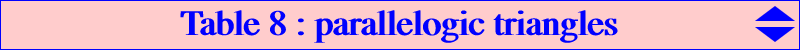Let P be a point in the plane of the reference triangle ABC and denote by cevP : the cevian triangle of P acvP : the anticevian triangle of P pedP : the pedal triangle of P apdP : the antipedal triangle of P ccvP : the circumcevian triangle of P cacP : the circumanticevian triangle of P refP : the reflection triangle of P in the sidelines of ABC symP : the reflection triangle of P in the vertices of ABC The following table gives the loci of P such that two of these triangles are parallelogic. Note the frequent occurence of the Kjp cubic K024 and see Table 22 for a generalization. true means the property is true for any P, L denotes the line at infinity, C denotes the circumcircle.ABC cevP acvP pedP apdP ccvP cacP refP cevP sidelines of ABC and antimedial triangle acvP sidelines of ABC sidelines of ABC and a cubic pedP L, C L, C and the quartic Q1 C and a quartic apdP L, C L, C and a quintic L, sidelines of ABC and polar circle L, C and K024 ccvP L, C and K024 C and a septic sidelines of ABC, C and a bicircular quartic L, C and K024 L, C cacP a sextic a nonic sidelines of ABC L, the quartic Q2 and a nK(X6, X3) L, C and an octic C and the quartic Q2 refP L, C C and the quartic Q1 C and a quartic L, C L, C and K024 L, C and K024 C, the quartic Q2 and K003 symP not possible sidelines of the antimedial triangle sidelines of ABC L L, C L, C and K024 a sextic LNotes : the quartic Q1 is the isogonal transform of a conic. the quartic Q2 is the isogonal transform of the polar circle.